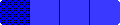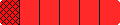Home

# Equations Game

 In this game you will be dealt 10 Fraction Bars or 10 Fraction Playing Cards. The object is to drag the fractions into place to form as many equations as you can. When you have formed your equations click GO to check the equations and obtain your score. You will receive one point for each fraction in a correct equation. There are three rounds of 10 fractions each and you win the game if you score a total of 21 or more points.